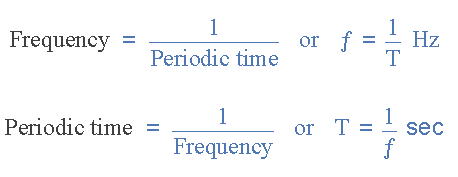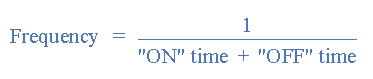# Describe Different types of Electrical Waveforms.

## Electrical Waveforms

In our previous tutorials of  Oscillator , we saw that an oscillator is an electronic circuit used to generate a continuous output signal usually in the form of a sinusoid at some predetermined frequency or wavelength which is set by the resonant components of the circuit.

We also saw that there are many different types of oscillator circuits available but generally they all consist of an amplifier and either an Inductor-Capacitor, ( LC ) or Resistor-Capacitor, ( RC ) tank circuit used to produce a sine wave type output signal.

But sometimes in electronic circuits we need to produce many different types, frequencies and shapes of Signal Waveforms such as Square Waves, Rectangular Waves, Triangular Waves, Sawtoothed Waveforms and a variety of pulses and spikes.

These types of signal waveform are used for either timing signals, clock signals or as trigger pulses.

In this article we will understand the basic characteristics that make up Electrical Waveforms.

Electrical Waveforms are basically visual representations of the variation of a voltage or current over time.

In simple language if we plot on a piece of graph paper these voltage or current variations  on x- axis against  time (t ) on y- axis , the resulting plot or drawing would represent the shape of a Waveform .

There are many different types of electrical waveforms available but generally they can all be broken down into two distinctive groups.

1. Uni-directional Waveforms : These electrical waveforms are always positive or negative in nature flowing in one forward direction only and they never cross the zero axis point. Common uni-directional waveforms are Square-wave timing signals, Clock pulses and Trigger pulses.
2. Bi-directional Waveforms : These electrical waveforms are also called alternating waveforms as they alternate from a positive direction to a negative direction constantly crossing the zero axis point. Bi-directional waveforms go through periodic changes in amplitude. The most common bi-directional waveform is the Sine-wave.

Whether the waveform is uni-directional/bi-directional, periodic/non-periodic, symmetrical/non-symmetrical, simple/complex, all electrical waveforms include the following three common characteristics:

1. Period: – This is the length of time in seconds that the waveform takes to repeat itself from start to finish. This value can also be called the Periodic Time, ( T ) of the waveform for sine waves, or the Pulse Width for square waves.
2. Frequency: – This is the number of times the waveform repeats itself within a one second time period. Frequency is the reciprocal of the time period, ( ƒ = 1/T ) with the standard unit of frequency being the Hertz, (Hz).
3. Amplitude: – This is the magnitude or intensity of the signal waveform measured in volts or amps.

### Periodic Waveforms

Periodic waveforms are the most common of all the electrical waveforms and it includes Sine Waves.

The AC (Alternating Current) mains waveform in your home is a sine wave and it constantly alternates between a maximum value and a minimum value over time.

The amount of time it takes for the waveform to repeat itself is known as its “periodic time” or simply the Period of the waveform.

This period can vary with each waveform from fractions of a second to thousands of seconds as it depends upon the frequency of the waveform.

For example, a sinusoidal waveform which takes one second to complete its cycle will have a periodic time of one second. Likewise a sine wave which takes five seconds to complete will have a periodic time of five seconds and so on.

### Sine Wave Waveform

If we take the reciprocal of the period, ( 1/T ) we will get a value that denotes the number of times a period or cycle repeats itself in one second or cycles per second, and this is commonly known as Frequency with units of Hertz, (Hz). Then Hertz can also be defined as “cycles per second” (cps) and 1Hz is exactly equal to 1 cycle per second.

### Relationship between Frequency and Periodic TimeWhere:  ƒ is in Hertz and T is in Seconds.

### Square Wave Electrical Waveforms

Square-wave Waveforms are used extensively in electronic and micro electronic circuits for clock and timing control signals as they are symmetrical waveforms of equal and square duration representing each half of a cycle. Nearly all digital logic circuits use square wave waveforms on their input and output gates.

### A Square Wave Waveform

We know that square shaped electrical waveforms are symmetrical in shape as each half of the cycle is identical, so the time that the pulse width is positive must be equal to the time that the pulse width is negative or zero.

When square wave waveforms are used as “clock” signals in digital circuits the time of the positive pulse width is known as the “Duty Cycle” of the period.

Then we can say that for a square wave waveform the positive or “ON” time is equal to the negative or “OFF” time so the duty cycle must be 50%, (half of its period).

As frequency is equal to the reciprocal of the period, ( 1/T ) we can define the frequency of a square wave waveform as:### Example:

A Square Wave electrical waveform has a pulse width of 10 ms, calculate its frequency, ( ƒ ).

#### Solution:

For a square wave shaped waveform, the duty cycle is given as 50%, therefore the period of the waveform must be equal to: 10 ms + 10 ms = 20 ms

So frequency , f = 1/Period = 1/20 ms = 50 Hz

### Rectangular Waveforms

Rectangular Waveforms are similar to the square wave waveform above, only the difference is that the two pulse widths of the waveform are of an unequal time period.

Rectangular waveforms are therefore classed as “Non-symmetrical” waveforms as shown below.

The example above shows that the positive pulse width is shorter in time than the negative pulse width.

Equally, the negative pulse width could be shorter than the positive pulse width, either way the resulting waveform shape would still be that of a rectangular waveform.

These positive and negative pulse widths are sometimes called “Mark” and “Space” respectively, with the ratio of the Mark time to the Space time being known as the “Mark-to-Space” ratio of the period and for a Square wave waveform this would be equal to one.

### Example:

A Rectangular waveform has a positive pulse width (Mark time) of 10 ms and a duty cycle of 25%, calculate its frequency.

#### Solution:

The duty cycle is given as 25% or 1/4 and this is equal to the mark time which is 10ms,

then the period of the waveform must be equal to:

10 ms (25%) + 30 ms (75%) = 40 ms (100%)

So frequency , f = 1/Period = 1/40 ms = 25 Hz

Rectangular Waveforms can be used to regulate the amount of power being applied to a load such as a lamp or motor by varying the duty cycle of the waveform.

The higher the duty cycle, the greater the average amount of power being applied to the load and the lower the duty cycle, the less the average amount of power being applied to the load .

An excellent example of this is in the use of “Pulse Width Modulation” speed controllers.

### Triangular Waveforms

Triangular Waveforms are generally bi-directional non-sinusoidal waveforms that oscillate between a positive and a negative peak value.

Although called a triangular waveform, the triangular wave is actually more of a symmetrical linear ramp waveform because it is simply a slow rising and falling voltage signal at a constant frequency or rate.

The rate at which the voltage changes between each ramp direction is equal during both halves of the cycle as shown below.

Generally, for Triangular Waveforms the positive-going ramp or slope (rise), is of the same time duration as the negative-going ramp (decay) giving the triangular waveform a 50% duty cycle.

Then any given voltage amplitude, the frequency of the waveform will determine the average voltage level of the wave.

So for a slow rise and slow delay time of the ramp will give a lower average voltage level than a faster rise and decay time.

However, we can produce non-symmetrical triangular waveforms by varying either the rising or decaying ramp values to give us another type of waveform known commonly as a Sawtooth Waveform.

### Sawtooth Waveforms

Sawtooth Waveforms are another type of periodic waveform. As its name suggests, the shape of the waveform resembles the teeth of a saw blade.

Sawtoothed waveforms can have a mirror image of themselves, by having either a slow-rising but extremely steep decay, or an extremely steep almost vertical rise and a slow-decay as shown below.

The positive ramp Sawtooth Waveform is the more common of the two waveform types with the ramp portion of the wave being almost perfectly linear.

The Sawtooth waveform is commonly available from most function generators and consists of a fundamental frequency ( ƒ ) and all its integer ratios of even harmonics only, 1/2, 1/4, 1/6 1/8 … 1/n etc.

What this means in practical terms is that the Sawtoothed Waveform is rich in harmonics and for music synthesizers and musicians gives the quality of the sound or tonal colour to their music without any distortion.

### Triggers and Pulses

Although technically Triggers and Pulses are two separate waveforms, we can combine them together here, as a “Trigger” is basically just a very narrow “Pulse”.

The difference being is that a trigger can be either positive or negative in direction whereas a pulse is only positive in direction.

A Pulse Waveform or “Pulse-train” as they are more commonly called, is a type of non-sinusoidal waveform that is similar to the Rectangular waveform we discussed earlier.

The difference is that the exact shape of the pulse is determined by the “Mark-to-Space” ratio of the period and for a pulse or trigger waveform the Mark portion of the wave is very short with a rapid rise and decay shape as shown below.

### A Pulse Waveform

The purpose of a “Pulse” and that of a trigger is to produce a very short signal to control the time at which something happens for example, to start a Timer, Counter, Monostable or Flip-flop etc, or as a trigger to switch “ON” SCRs(Thyristors), Triacs  and other power semiconductor devices.

### Function Generator

A Function Generator or Waveform Generator is a device or circuit that produces a variety of different waveforms at a desired frequency.

It can generate Sine waves, Square waves, Triangular and Sawtooth waveforms as well as other types of output waveforms.

There are many “off-the-shelf” waveform generator IC’s available and all can be incorporated into a circuit to produce the different periodic waveforms required.

One such device is the 8038 a precision waveform generator IC capable of producing sine, square and triangular output waveforms, with a minimum number of external components or adjustments. Its operating frequency range can be selected over eight decades of frequency, from 0.001Hz to 300kHz, by the correct choice of the external R-C components.

### Waveform Generator IC

The frequency of oscillation is highly stable over a wide range of temperature and supply voltage changes and frequencies as high as 1MHz is possible.

Each of the three basic waveform outputs, sinusoidal, triangular and square are simultaneously available from independent output terminals.

The frequency range of the 8038 is voltage controllable but not a linear function. The triangle symmetry and hence the sine wave distortion are adjustable.

In the next tutorial about Waveforms, we will discuss Multivibrators that are used to produce continuous output waveforms or single individual pulses. One such multivibrator circuit that is used as a pulse generator is called a Monostable Multivibrator.